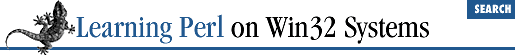home | O'Reilly's CD bookshelfs | FreeBSD | Linux | Cisco | Cisco Exam

### 3.8 Exercises

1. Write a program that reads a list of strings on separate lines and prints out the list in reverse order. If you're reading the list from the console, you'll probably need to delimit the end of the list by pressing CTRL-Z.

2. Write a program that reads a number and then a list of strings (all on separate lines), and then prints one of the lines from the list as selected by the number.

3. Write a program that reads a list of strings and then selects and prints a random string from the list. To select a random element of ``` @somearray``` , put

`     srand;`

at the beginning of your program (this initializes the random number generator), and then use

```

rand(@somearray)```

where you need a random value between 0 and 1 less than the length of``` @somearray``` .3.7 Variable Interpolation of Arrays4. Control Structures